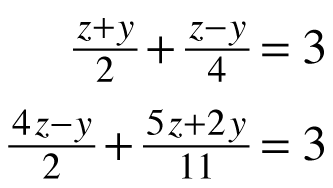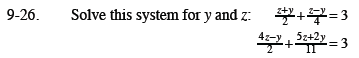Home > CCA2 > Chapter 9 > Lesson 9.1.2 > Problem9-26

9-26.

Solve this system for y and z: Homework Help ✎Simplify the equations before solving.

Multiply both sides by 4.

$4\left( \frac{z+y}{2}+\frac{z-y}{4} \right) =4\cdot3$

Simplify.

$2(z+y)+z-y =12$

$2z+2y+z-y =12$

$3z+y=12$

Now do the same for the 2nd equation. But multiply both sides by 22. When both equations are simplifed, solve for z and y.